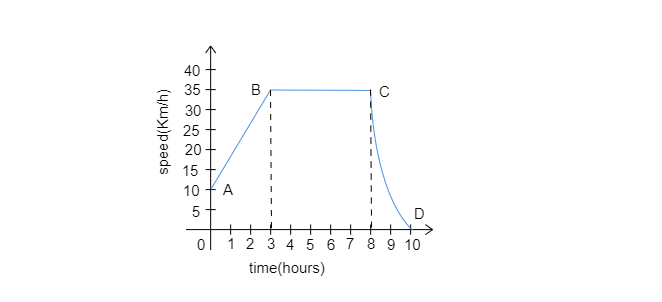(i) What is the initial speed of the car?
(ii) What is the maximum speed attained by the car?
(iii) Which part of the graph shows zero acceleration?
(iv) Which part of the graph shows varying retardation?
(v) Find the distance travelled in first 8 hours."
">

# The graph given alongside shows how the speed of a car changes with time:(i) What is the initial speed of the car?(ii) What is the maximum speed attained by the car?(iii) Which part of the graph shows zero acceleration?(iv) Which part of the graph shows varying retardation?(v) Find the distance travelled in first 8 hours."

(i). As the slope of the graph starts from point A which indicates $10\ km/h$. So, the initial speed of the car is $10\ km/h$.

(ii). The slope of the given speed-time graph of the car is maximum at $35\ km/h$. so, $35\ km/h$ is the maximum speed attained by the car.

(iii). In parts B to C, the speed remains constant. So, part BC shows zero acceleration.

(iv). In the given graph, speed decreases from C to D. So, the part CD shows varying retardation.

(v). The distance traveled in the first $8\ hours$ is equal to the area under AB and BC.

So, the distance traveled by car $=$Area of triangle$+$Area of the rectangle

$=\frac{1}{2}\times(35-10)(3)+35\times(8-3)$

$=37.5+175$

$=212.5\ km/h$

Updated on: 10-Oct-2022

651 Views